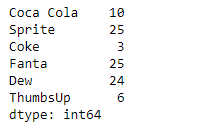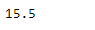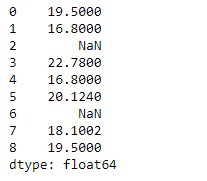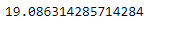# Python | Pandas Series.mean()

Pandas series is a One-dimensional ndarray with axis labels. The labels need not be unique but must be a hashable type. The object supports both integer- and label-based indexing and provides a host of methods for performing operations involving the index.

Pandas` Series.mean()` function return the mean of the underlying data in the given Series object.

Syntax: Series.mean(axis=None, skipna=None, level=None, numeric_only=None, **kwargs)

Parameter :
axis : Axis for the function to be applied on.
skipna : Exclude NA/null values when computing the result.
level : If the axis is a MultiIndex (hierarchical), count along a particular level, collapsing into a scalar.
numeric_only : Include only float, int, boolean columns.
**kwargs : Additional keyword arguments to be passed to the function.

Returns : mean : scalar or Series (if level specified)

Example #1: Use `Series.mean()` function to find the mean of the underlying data in the given series object.

 `# importing pandas as pd ` `import` `pandas as pd ` ` `  `# Creating the Series ` `sr ``=` `pd.Series([``10``, ``25``, ``3``, ``25``, ``24``, ``6``]) ` ` `  `# Create the Index ` `index_ ``=` `[``'Coca Cola'``, ``'Sprite'``, ``'Coke'``, ``'Fanta'``, ``'Dew'``, ``'ThumbsUp'``] ` ` `  `# set the index ` `sr.index ``=` `index_ ` ` `  `# Print the series ` `print``(sr) `

Output :Now we will use `Series.mean()` function to find the mean of the given series object.

 `# return the mean ` `result ``=` `sr.mean() ` ` `  `# Print the result ` `print``(result) `

Output :As we can see in the output, the `Series.mean()` function has successfully returned the mean of the given series object.

Example #2: Use `Series.mean()` function to find the mean of the underlying data in the given series object. The given series object also contains some missing values.

 `# importing pandas as pd ` `import` `pandas as pd ` ` `  `# Creating the Series ` `sr ``=` `pd.Series([``19.5``, ``16.8``, ``None``, ``22.78``, ``16.8``, ``20.124``, ``None``, ``18.1002``, ``19.5``]) ` ` `  `# Print the series ` `print``(sr) `

Output :Now we will use `Series.mean()` function to find the mean of the given series object. we are going to skip all the missing values while calculating the mean.

 `# return the mean ` `# skip all the missing values ` `result ``=` `sr.mean(skipna ``=` `True``) ` ` `  `# Print the result ` `print``(result) `

Output :As we can see in the output, the `Series.mean()` function has successfully returned the mean of the given series object.

Whether you're preparing for your first job interview or aiming to upskill in this ever-evolving tech landscape, GeeksforGeeks Courses are your key to success. We provide top-quality content at affordable prices, all geared towards accelerating your growth in a time-bound manner. Join the millions we've already empowered, and we're here to do the same for you. Don't miss out - check it out now!

Previous
Next